# CREST Reasoning Olympiad Class 7 Sample Papers

## Syllabus:

Section 1: Series Completion and Inserting the Missing Character, Analogy and Classification, Blood Relations, Coding - Decoding, Direction Sense Test, Logical Venn Diagram, Alpha Numeric Sequence Puzzle, Number, Ranking and Time Sequence Test, Logical Sequence of Words, Alphabet Test, Mathematical Operations, Analytical Reasoning, Mirror Images and Water Images, Embedded Figures, Figure Formation, Construction of Squares, Grouping of Identical Figures, Figure Matrix, Paper Folding and Paper Cutting, Cubes and Dice, Dot Situations.

Achievers Section: Higher Order Thinking Questions - Syllabus as per Section 1

 Q.1 Select a suitable figure from the four alternatives that would complete the figure matrix.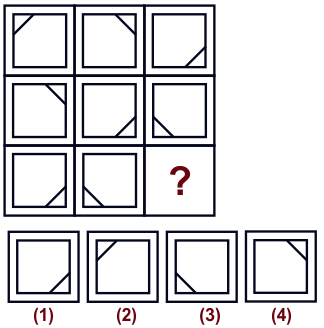Q.2 Choose a figure which would most closely resemble the unfolded form of Figure (Z).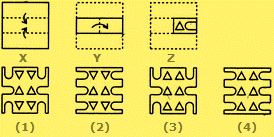Q.3 Find the best alternative among given that would appear as the pattern given when the transparent sheet is folded at the dotted line.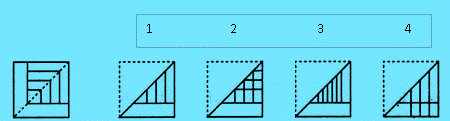Q.4 Choose a figure which would most closely resemble the unfolded form of Figure (Z).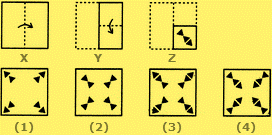Q.5 Find out from amongst the four alternatives (a), (b), (c), (d) - as to how the pattern would appear when the transparent sheet(X) is folded at the dotted line.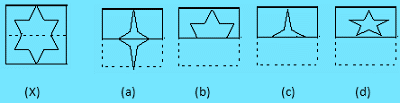Q.6 Find out the alternative figure which contains figure as its part.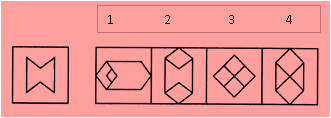Q.7 Find out the alternative figure which contains figure (X) as its part.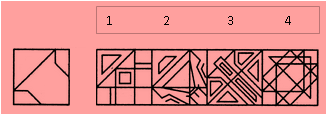Q.8 Find out the alternative figure which contains figure as its part.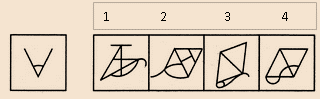Q.9 Choose a figure which would most closely resemble the unfolded form of Figure (Z).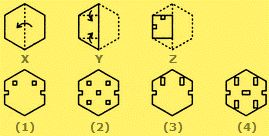Q.10 A dice is numbered from 1 to 6 in different ways. If 1 is adjacent to 2, 4 and 6, then which of the following statements is necessarily true?

Sample PDF of CREST Reasoning Olympiad for Class 7: